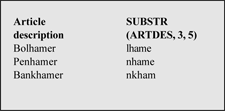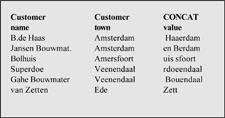# LANSA and SQL Tip: Scalar built in functions(2)

Date: Archived LANSA - All Platforms Few more handy examples of scalar built-in functions in SQL LANSA Netherlands

SQL consists of two types of built in functions:

Scalaire functions These are functions which can use only one value.

Column functions These are functions which can use a number of elements in a column

Here are a few examples of the scalaire built in functions SUBSTR, CONCAT, MAX and MIN.1)SUBSTR

Returns the selected part of a character string.

SUBSTR (expression, integer, integer)

The first integer is used to define the start position of the string, the second integer is optional and can be used to define the length to be extracted.

Example:

`FIELD = 'ASPECT COMPUTING (EUROPE)'SUBSTR(FIELD, 10, 5) = 'MPUTI'SUBSTR(FIELD, 18) = '(EUROPE)'SELECT_SQL    FIELDS((#ARTDES) (#SUBDES 'SUBSTR(ARTDES, 3, 5)'))    FROM_FILES((ARTMAST))ENDSELECT`2) CONCAT

Strings two values together

String CONCAT string

Example:

`FLDA CONCAT ‘ ‘ CONCAT FLDB`

The values of FLDA and FLDB are strung together with one blank between them

`SELECT_SQL    FIELDS(#TEXT50 'CUSNAM CONCAT'' ''CONCAT CUSTWN')    FROM_FILES((CUSMAST))ENDSELECT`

It is also possible to combine SUBSTR:

`SELECT_SQL    FIELDS((#CUSNAM) (#CUSTWN) (#HLPVAR 'SUBSTR(CUSNAM, 5, 4) CONCAT SUBSTR(CUSTWN, 5, 8)'))    FROM_FILES((CUSMAST))ENDSELECT`

3) MAX

Returns the highest value of a range of given fields - at least two fields must be defined

MAX (expression, expression, etc)

Example:

MAX(VALUE1,VALUE2, VALUE3)

When VALUE1 = 100,00

and VALUE2 = 35,75

and VALUE3 = 54,20

Returned value = 100,00.

Example:

MAX(FLDA, FLDB)

Value of FLDA = JANSEN

Value of FLDB = AALBERS

Returned value = JANSEN.

4) MIN

The opposite of MAX

If the same examples as above are used and MAX is replaced by MIN, the returned values are 35,75 and AALBERS.

Return the record with the highest and lowest price:

`SELECT_SQL    FIELDS((#MIN ‘MIN(ARTPRS)') (#MAX ‘MAX(ARTPRS)'))    FROM_FILES((ARTMAST))ENDSELECT`